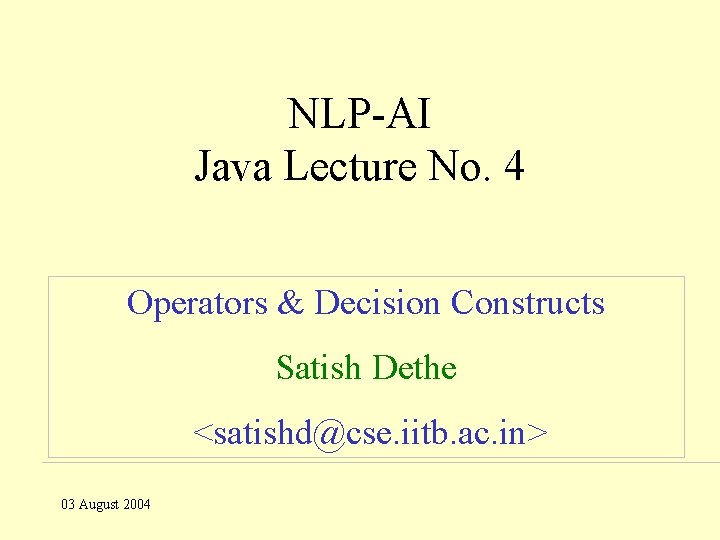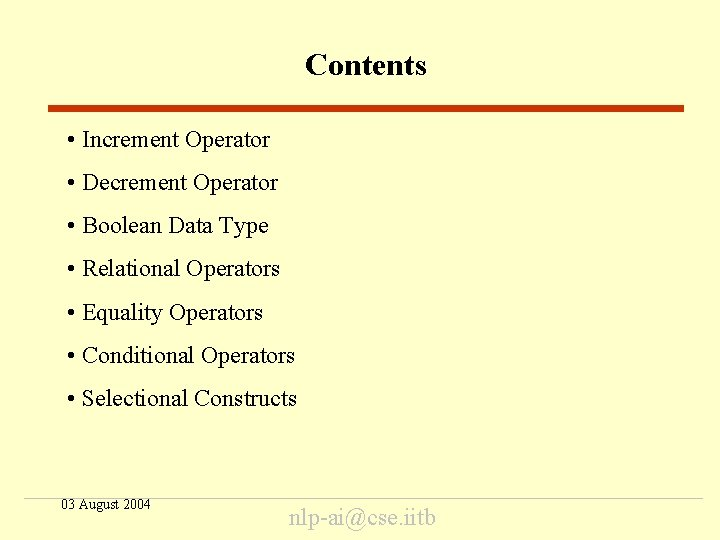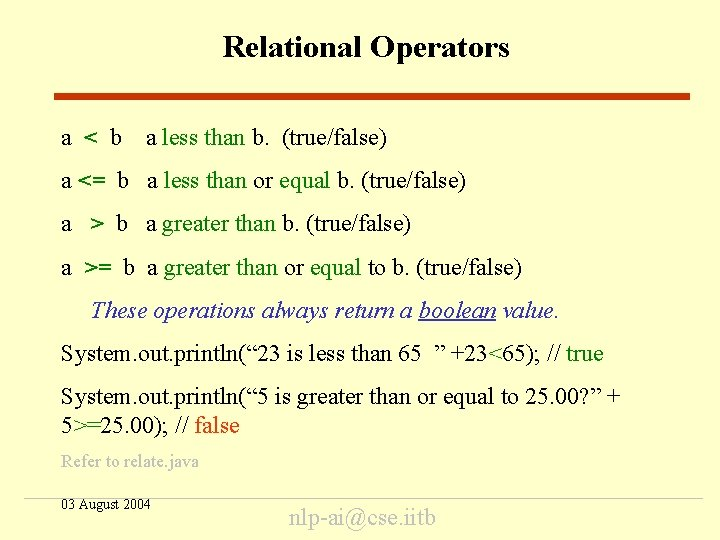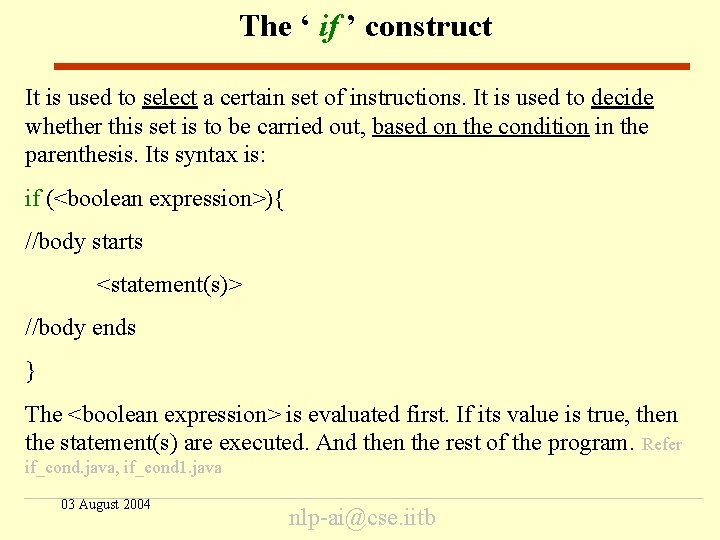# NLPAI Java Lecture No 4 Operators Decision Constructs

• Slides: 12NLP-AI Java Lecture No. 4 Operators & Decision Constructs Satish Dethe <[email protected] iitb. ac. in> 03 August 2004Contents • Increment Operator • Decrement Operator • Boolean Data Type • Relational Operators • Equality Operators • Conditional Operators • Selectional Constructs 03 August 2004 [email protected] iitbIncrement Operators • i + + first use the value of i and then increment it by 1. ‘i + +’ equivalent to ‘i = (i)+1’. // postfix increment • + + i first increment the value of i by 1 and then use it. ‘+ + i’ equivalent to ‘i = (i+1)’. // prefix increment i=2; • System. out. print(“ i = ”+ i++); //print 2, then i becomes 3 • System. out. print(“ i = ”+ ++i); //add 1 to i, then print 4 • Refer to incre. java 03 August 2004 [email protected] iitbDecrement Operators • i - - first use the i ’s value and then decrement it by one i - - equivalent to (i)-1. // postfix decrement • - - i first decrement i ’s value by one and then use it. - - i equivalent to (i-1). // prefix decrement i=5; System. out. print(“i = ” + i--); //print 5, then i becomes 4 System. out. print(“i = ” + --i); //subtract 1 from i, then print 3 Refer to decre. java 03 August 2004 [email protected] iitbBoolean Data Type This data type can store only two values; true and false. Declaring a boolean variable is the same as declaring any other primitive data type like int, float, char. boolean response = false; //Valid answer = true; //Valid answer = 9943; //Invalid, response = “false”; // Invalid, This is return type for relational & conditional operators. Refer to bool_op. java 03 August 2004 [email protected] iitbRelational Operators a < b a less than b. (true/false) a <= b a less than or equal b. (true/false) a > b a greater than b. (true/false) a >= b a greater than or equal to b. (true/false) These operations always return a boolean value. System. out. println(“ 23 is less than 65 ” +23<65); // true System. out. println(“ 5 is greater than or equal to 25. 00? ” + 5>=25. 00); // false Refer to relate. java 03 August 2004 [email protected] iitbEquality Operators a==b a equal to b. (true/false) a!=b a not equal to b. (true/false) boolean equal = 12 = = 150; // false boolean again_equal = ‘r’= = ‘r’); // true boolean not_equal = 53!=90); // true Refer: equa. java 03 August 2004 [email protected] iitbConditional Operators • A conditional operator is used to handle only two boolean expressions. Boolean expression always returns ‘true’ or ‘false’. • Conditional AND ‘&&’ Return value is ‘true’ if both, x and y are true, else it is ‘false’. System. out. println(“x&&y ” + x&&y); // Refer cond_and. java • Conditional OR ‘||’ return value is true if any one of x or y, is true else it is false. System. out. println(“x||y ” + x||y); // Refer cond_or. java 03 August 2004 [email protected] iitbThe ‘ if ’ construct It is used to select a certain set of instructions. It is used to decide whether this set is to be carried out, based on the condition in the parenthesis. Its syntax is: if (<boolean expression>){ //body starts <statement(s)> //body ends } The <boolean expression> is evaluated first. If its value is true, then the statement(s) are executed. And then the rest of the program. Refer if_cond. java, if_cond 1. java 03 August 2004 [email protected] iitbThe ‘if else’ construct It is used to provide an alternative when the expression in if is false. Its syntax is: if(<boolean expression>){ <statement(s)> } else{ <statement(s)> } The if construct is the same. But when the expression inside if is false then else part is executed. Refer ifelse_cond. java 03 August 2004 [email protected] iitbAssignments int a_number=1; // (range: 1 to 5 including both) Print the value of a_number in word. For example, it should print “Four” if a_number contains 4. 1. 2. Use equality ‘= =’ operator. Do not use equality ‘= =’ operator. 03 August 2004 [email protected] iitbEnd Thank You! 03 August 2004 [email protected] iitb